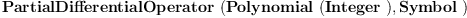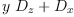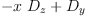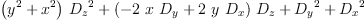login  home  contents  what's new  discussion  bug reports help  links  subscribe  changes  refresh  edit

FriCAS can compute Groebner bases for noncommutative polynomial rings of solvable type (of category SolvableSkewPolynomialCategory?). Below we give example using partial differential operators:

fricas
(1) -> Pdo := PartialDifferentialOperator(Polynomial(Integer), Symbol)(1)
Type: Type
fricas
xx := D(x)$Pdo + y*D(z)$Pdo(2)
Type: PartialDifferentialOperator?(Polynomial(Integer),Symbol)
fricas
yy := D(y)$Pdo - x*D(z)$Pdo(3)
Type: PartialDifferentialOperator?(Polynomial(Integer),Symbol)
fricas
L := xx*xx + yy*yy(4)
Type: PartialDifferentialOperator?(Polynomial(Integer),Symbol)
fricas
gPak := NGroebnerPackage(Polynomial(Integer), IndexedExponents(Symbol), Symbol, Pdo)
The constructor NGroebnerPackage takes 3 arguments and you have
given  4  .

 Subject:   Be Bold !! ( 14 subscribers )